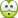# Finding the center of instantanous rotation

• Engineering
Poster has been reminded to show their work on schoolwork problems

## Homework Statement:

Look at the image

## Relevant Equations:

v=w.r
[Mentor Note -- Improved versions of the two pictures are posted in a reply a few posts down]

Good dayand here is the solutionI have a problem in finding the value of AC and BC, I couldn't figure it out?

Last edited by a moderator:
•Delta2

Related Engineering and Comp Sci Homework Help News on Phys.org
BvU
Homework Helper
I have a problem in finding the value of AC and BC, I couldn't figure it out?
I don't think your relevant equation is going to help you there. Don't you have anything else in your toolbox ? Something geometrical or trigonometrical, perhaps ? Keep searching !

ah, from the poor picture I can see they resort to geometry !
Free tip: photograph from above, or better: rendere your own drawing and post !

it's certainly something geometric but I couldn't figure it out

berkeman
Mentor
Here are improved versions of the OP's dark pictures:•Lnewqban and Amaelle
BvU
Homework Helper
it's certainly something geometric but I couldn't figure it out
I made a drawing and needed the coordinates of D. From the picture it isn't all that clear but I guess you can check in your book that (180,0) isn't all that bad...
And what about B?

With that settled, things are obvious, right ?

not reallycan you please be more explicit?

BvU
Homework Helper
My post was actually a question:

Can you confirm that O and D are at the same y-coordinate in your book ? And B and D at the same x ?

To become even more explicit:
Long ago, I learned about Pythagoras. The numbers 3,4,5 and 5,12,13 are still hanging around in my head ...

•berkeman and Amaelle
yes I can confirm that O and D are at the same y-coodinate And B and D at the same x

BvU
Homework Helper
With that settled, things are obvious, right ?
So what is the perpendicular distance from A to BD ?

60+180=240

BvU
Homework Helper
So do you now see the 3,4,5 and 5,12,13 rectangular triangles ?

so let's consider A' as the projection of A on BD
you are talking about the triangles AA'B and AA'C
we know all the values of the triangle AA'B but we only know one value the length AA' for the triangle AA'C
but I still can't see how can we apply that phytagora theorem (except from finding BA')
thanks a lot!

BvU
Homework Helper
AD is the hypothenuse of a 3,4,5 triangle. So AA'C is also a 3,4,5 triangle•Amaelle
so, if I understood well, you are talking about a rule of two rectangular triangles one inside the other , you called 3,4,5 and 5, 12 , 13 , I tried to google it and only find something regarding Fibonacci numbers in Pythagorean triples
I would be very grateful if you give me a link to see that rule

BvU
Homework Helper
$$3^2+4^2=5^2$$is known, right ?Since the two red angles are the same, AA' is the 3 of 3,4,5 in AA'C

•berkeman, Lnewqban and Amaelle
thank you but the attachement does not open!

BvU
Homework Helper
thank you but the attachement does not open!
I re-did it. Should be a picture now. But you have your own picture too, right ?

•Amaelle
thanks a million! it's clear now
best regards!

•Lnewqban, berkeman and BvU
BvU
The exercise is very artificial, but I enjoyed helping•